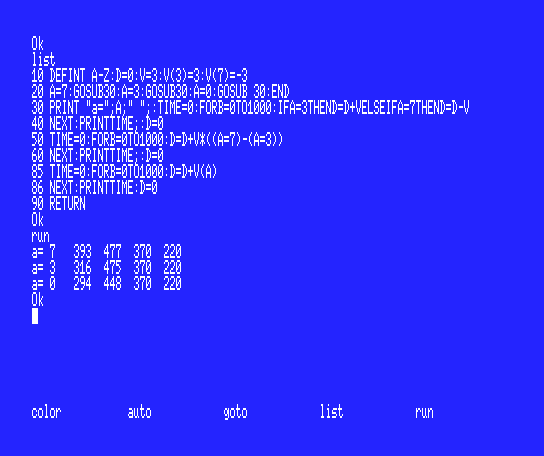# TFH's new project - Shooting Stars 2022 - Help Wanted

Page 3/4
1 | 2 | | 4
tfh wrote:
Quote:

Also slightly faster version of IFA=3THEND=D+VELSEIFA=7THEND=D-V is to write it as
D=D+V*(-(A=3)+(A=7))

Are you sure?

Actually nobut there must have been a reason why I did it like that in the past when I did use BASIC.
So I tested it again using this program.

```10 DEFINT A-Z:D=0:V=3
20 A=7:GOSUB30:A=3:GOSUB30:A=0:GOSUB 30:END
30 PRINT "a=";A;" ";:TIME=0:FORB=0TO1000:IFA=3THEND=D+VELSEIFA=7THEND=D-V
40 NEXT:PRINTTIME;:D=0
50 TIME=0:FORB=0TO1000:D=D+V*((A=7)-(A=3))
60 NEXT:PRINTTIME;:D=0
70 TIME=0:FORB=0TO1000:D=D+(A=7)-(A=3)
80 NEXT:PRINTTIME
90 RETURN
```

And the output was
a= 7 332 403 312
a= 3 267 401 312
a= 0 249 378 313

So I conclude that
a) my method is indeed slower but always uses a constant time while the if method varies depending on input
b) the impact of the multiplication is significant, almost 25% extra time needed

tfh wrote:
Quote:

Now for the sprite issues.

The thing is that I actually do set the sprites after each page swap.

```530 SPRITE\$(0)=CHR\$(16)+CHR\$(56)+CHR\$(124)+CHR\$(254)+CHR\$(124)+CHR\$(56)+CHR\$(16)
540 SPRITE\$(1)=CHR\$(129)+CHR\$(129)+CHR\$(129)+CHR\$(195)+CHR\$(165)+CHR\$(153)+CHR\$(255)+CHR\$(255)
550 RETURN```

Jipe found a workaround, but I wonder if doing a 'screen,0' before the first SPRITE\$(0) and setting the colors with COLOR SPRITE\$(0)=STRING\$(8,7):COLOR SPRITE\$(1)=STRING\$(8,6) would help?

`360 IFA=3THEND=D+VELSEIFA=7THEND=D-V`

When setting speed in level settings rows, use an array instead of a single V variable:
`V(3)=3:V(7)=-3`

On this way then you can do:

`360 D=D+V(A)`

So, when A=3 (so stick(0)=3) you have D=D+V(3) so D=D+3
When A=7 you have D=D+A(7) so D=D+(-3) so D=D-3

Faster and shorter (so even more faster)

tfh wrote:
gdx wrote:

And change page with the VDP instruction instead of SET PAGE?

Sure, I can try that. I didn't know you can also do it with a VDP command. Which would that be?

In SCREEN 5, You can try with:

• POKE&HFAF5,x: VDP(2)=x*32+31 instead of SETPAGE x

Not sure but I think it's better to use the POKE and VDP without graphic instruction that follow on a line.

Obviously, before test if is SETPAGE x or SETPAGE ,x which causes the issue.
You can directly put the calculation result value for VDP(2) instruction. (&H1F, &H3F, &H5F or &H7F)

Wow! Thank you all for your input! I've got some things to test / change / update. I'm learning a lot again. Thanks!
The next days I will be helping moving my daughter to a new house, so I don't know when exactly I can try all of the above, but.. I have some homework to do.

Thanks again!

The way BASIC abstracts the VDP table address registers as pages is kinda non-obvious, so when you change pages it can still show some uninitialized garbage on the screen, especially when it comes down to sprites, so I'd suggest you doing a CLS before you start using a page (lines 210-240 in the first listing you showed).

POKE&HFAF5,x: VDP(2)=x*32+31 instead of SETPAGE x
i have testing this method but in level 2 the sprite of ship no move and no star on screen
but the star counter at the right is incremented

Parn, are you sure CLS has an action on sprites?

I wrongly assumed CLS initialized the sprite area, but unfortunately it doesn't.So you would have to manually initialize the sprite area, I believe. This code can do it:

`FOR I=0 to 127 STEP 4:VPOKEBASE(28)+I,217:NEXT`

This would make every sprite invisible by default, and theoretically it wouldn't matter what's on the rest of the SAT.

Indeed... The POKING instead of Set Page didn't work out. CSL did neither and the VPOKES still gives me the same problem. Only creating the sp.bin file Jipe proposed seems to solve it. But if I need to have disk-access anyway, I might consider just loading the stages one by one (and maybe add a loading screen with some information of the upcoming level).
I was hoping that I had a small oversight, but it seems to be some weird MSX Basic behavior.

The way of controlling the spaceship thegeps proposed is indeed a lot quicker and even more stable in time:So I have updated that. I also found a bug that caused the number of stars remaining to be printed at the star position in the spacequake stages. Anyway, the updated version can be found here: https://test.file-hunter.com/
The sprite issue isn't implemented in this version, as I am going to move to loading the stages on the go.

Thank you all so far for thinking with me :)
My request for music remains though!

I think I solved the sprite issue. I looked into it more carefully and now I believe the problem is in the uninitalized sprite color table. It seemed to go away if I added COLORSPRITE(0)=0:COLORSPRITE(1)=0 before redefining the sprites on OpenMSX, but in WebMSX it seemed to need something like FORXX=0to31:COLORSPRITE(XX)=0:NEXTXX. I hope this also works on real hardware.

Page 3/4
1 | 2 | | 4